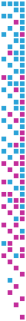# Gradient Estimation in Volume Data using 4D Linear Regression

László Neumann, Balázs Csebfalvi, Andreas König, Meister Eduard Gröller
Gradient Estimation in Volume Data using 4D Linear Regression
TR-186-2-00-03, February 2000

## Information

• Publication Type: Technical Report
• Workgroup(s)/Project(s): not specified
• Date: February 2000
• Number: TR-186-2-00-03
• Keywords: Linear Regression., Gradient Estimation, Volume Rendering

## Abstract

In this paper a new gradient estimation method is presented which is based on linear regression. Previous contextual shading techniques try to fit an approximate function to a set of surface points in the neighborhood of a given voxel. Therefore, a system of linear equations has to be solved using the computationally expensive Gaussian elimination. In contrast, our method approximates the density function itself in a local neighborhood with a 3D regression hyperplane. This approach also leads to a system of linear equations but we will show that it can be solved with an efficient convolution. Our method provides at each voxel location the normal vector and the translation of the regression hyperplane which are considered as a gradient and a filtered density value respectively. Therefore, this technique can be used for surface smoothing and gradient estimation at the same time.

No further information available.

## BibTeX

```@techreport{Csebfalvi-2000-GELR,
title =      "Gradient Estimation in Volume Data using 4D Linear
Regression",
author =     "L\'{a}szl\'{o} Neumann and Bal\'{a}zs Csebfalvi and Andreas
K\"{o}nig and Meister Eduard Gr\"{o}ller",
year =       "2000",
abstract =   "In this paper a new gradient estimation method is presented
which is based on linear regression. Previous contextual
shading techniques try to fit an approximate function to a
set of surface points in the neighborhood of a given voxel.
Therefore, a system of linear equations has to be solved
using the computationally expensive Gaussian elimination. In
contrast, our method approximates the density function
itself in a local neighborhood with a 3D regression
hyperplane.   This approach also leads to a system of linear
equations but we will show that it can be solved with an
efficient convolution. Our method provides at each voxel
location the normal vector and the translation of the
regression hyperplane which are considered as a gradient and
a filtered density value respectively. Therefore, this
technique can be used for surface smoothing and gradient
estimation at the same time. ",
month =      feb,
number =     "TR-186-2-00-03",
address =    "Favoritenstrasse 9-11/E193-02, A-1040 Vienna, Austria",
institution = "Institute of Computer Graphics and Algorithms, Vienna
University of Technology ",
note =       "human contact: technical-report@cg.tuwien.ac.at",
keywords =   "Linear Regression., Gradient Estimation, Volume Rendering",
URL =        "https://www.cg.tuwien.ac.at/research/publications/2000/Csebfalvi-2000-GELR/",
}
```